### Balanced Repeated Replication (BRR) Method

The balanced repeated replication (BRR) method requires that the full sample be drawn by using a stratified sample design with two primary sampling units (PSUs) per stratum. Let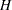be the total number of strata. The total number of replicates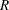is the smallest multiple of 4 that is greater than. However, if you prefer a larger number of replicates, you can specify the REPS=number option. If a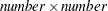Hadamard matrix cannot be constructed, the number of replicates is increased until a Hadamard matrix becomes available.

Each replicate is obtained by deleting one PSU per stratum according to the corresponding Hadamard matrix and adjusting the original weights for the remaining PSUs. The new weights are called replicate weights.

Replicates are constructed by using the firstcolumns of the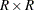Hadamard matrix. Theth (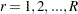) replicate is drawn from the full sample according to theth row of the Hadamard matrix as follows:

• If the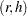th element of the Hadamard matrix is 1, then the first PSU of stratumis included in theth replicate and the second PSU of stratumis excluded.

• If theth element of the Hadamard matrix is –1, then the second PSU of stratumis included in theth replicate and the first PSU of stratumis excluded.

Note that the "first" and "second" PSUs are determined by data order in the input data set. Thus, if you reorder the data set and perform the same analysis by using BRR method, you might get slightly different results, because the contents in each replicate sample might change.

The replicate weights of the remaining PSUs in each half-sample are then doubled to their original weights. For more details about the BRR method, see Wolter (2007) and Lohr (2009).

By default, an appropriate Hadamard matrix is generated automatically to create the replicates. You can request that the Hadamard matrix be displayed by specifying the VARMETHOD=BRR(PRINTH) method-option. If you provide a Hadamard matrix by specifying the VARMETHOD=BRR(HADAMARD=) method-option, then the replicates are generated according to the provided Hadamard matrix.

You can use the VARMETHOD=BRR(OUTWEIGHTS=) method-option to save the replicate weights into a SAS data set.

Suppose that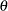is a population parameter of interest. Let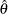be the estimate from the full sample for. Let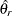be the estimate from theth replicate subsample by using replicate weights. PROC SURVEYMEANS  estimates the variance ofby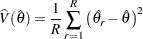withdegrees of freedom, whereis the number of strata.

If a parameter cannot be computed from one or more replicates, then the variance estimate is computed by using those replicates from which the parameter can be estimated. For example, suppose the parameter is a ratio. If a replicatecontains observations such that the denominator of the ratio is zero, then the ratio cannot be computed from replicate. In this case, the BRR variance estimate is computed as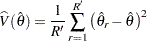where the summation is over the replicates where the parametercan be computed, and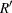is the number of those replicates.